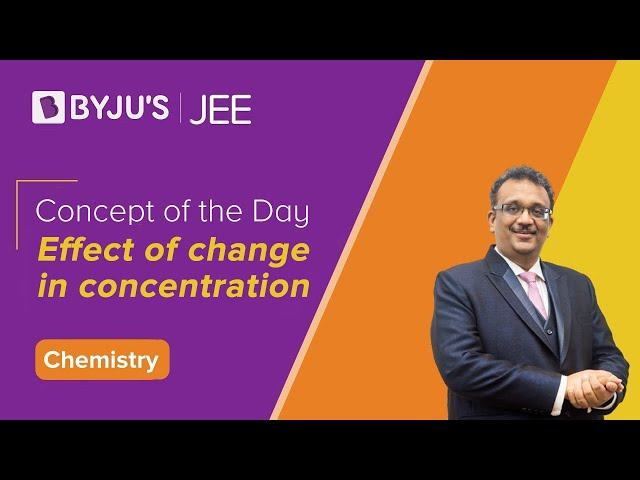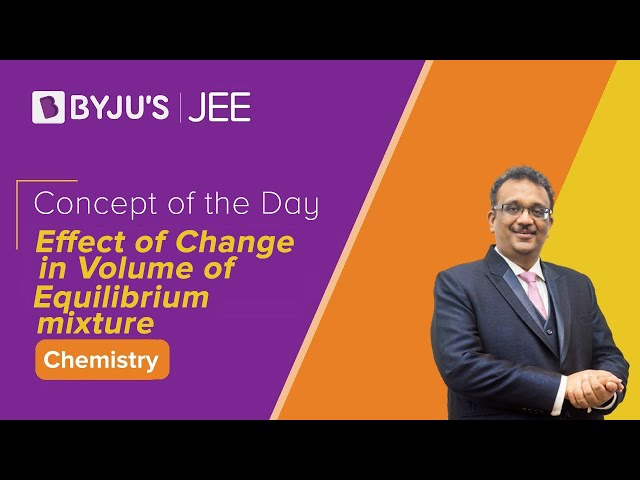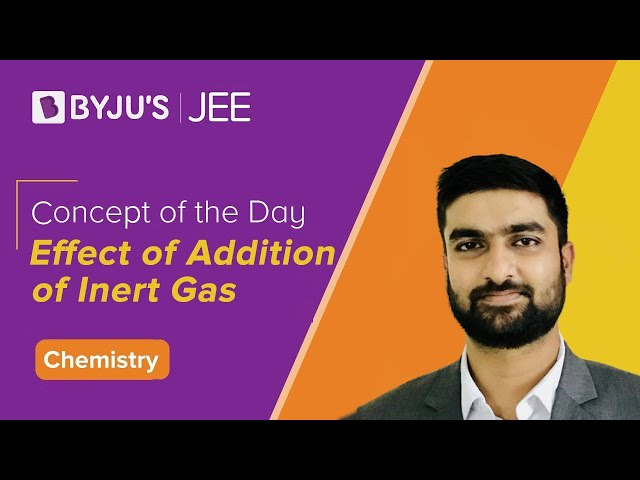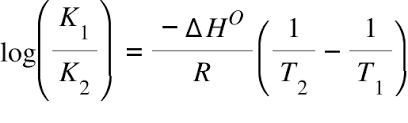# Le Chatelier's Principles on Equilibrium

## What are Le Chatelier’s Principles?

Le Chatelier’s principles, also known as the equilibrium law, are used to predict the effect of some changes on a system in chemical equilibrium (such as the change in temperature or pressure). The principle is named after the French chemist Henry Louis Le Chatelier.

Le Chatelier said that equilibrium adjusts the forward and backward reactions in such a way as to accept the changes affecting the equilibrium conditions.

### Table of Content

When factors like concentration, pressure, temperature, inert gases that affect equilibrium are changed, the equilibrium will shift in that direction where the effects caused by these changes are nullified.

Le Chatelier’s principles are often used to manipulate reversible reactions in order to obtain suitable outcomes (such as an improvement in yield).

### Effect of Concentration Changes on Equilibrium and Product Formation

As per Le Chatelier’s principles, the only way of equilibrium to accept more reactants is to increase product formation. The forward reaction is favoured when the concentration of the reactant is increased. The equilibrium of the reaction shift towards the use of reactants in the reaction, which decreases the concentration of the reactants.

Similarly, the addition of product (concentration/pressure) shall increase the backward reaction to decrease the product concentration. The backward reaction is favoured when the concentration of the reactant decreases and equilibrium of the reaction shift towards the production of reactants and the concentration of the reactants will be more.

Example:

Consider a reaction between oxygen and sulfur dioxide to produce sulfur trioxide.

2SO2(g) + O2(g) ⇋ 2SO3(g)

If the concentration of the reactant increases then

• Equilibrium will shift towards the decrease in the concentration of the reactants.
• More favoured for the forward reaction.
• Some of the  SO2 or O2 to form SO3.
• Equilibrium of the reaction shift towards the right.

If the concentration of the reactant decreases then

• Equilibrium will shift towards the increase in the concentration of the reactants.
• More favoured for the backward reaction.
• Some of the SO3would change to SO2 or O2.
• Equilibrium of the reaction shift towards the left.

If the product decreases then

• Equilibrium of the reaction shift to increases the concentration of the sulfur trioxide.
• Increase in the forward reaction rate.
• Some of the  SO2 or O2 to form SO3.
• Equilibrium of the reaction shift towards the right.

If the product increases then

• Equilibrium of the reaction shift to decrease the concentration of the sulfur trioxide.
• Increase in the reverse reaction rate.
• Some of the SO3would change to SO2 or O2.
• Equilibrium of the reaction shift towards the left.

#### Effect of Change in Concentration – Video Lesson### Effect of Change of Volume, Pressure, or Inert Gas on Equilibrium and Product Formation

Kp = Kc (RT)Δn = Kc (p/v)Δn

Change of volume, pressure or inert gases has no effect on the reactions of liquids and solids. They may have an effect in gaseous reactions and that too only when the difference in the sum of the number of reactant and product molecules (∆n) is not zero.

When ∆n = 0:

As per Le Chatelier’s principles, there will be no effect on Equilibrium and Product Formation on changing the volume, pressure or inert gas.

When ∆n = +ve:

An increase in pressure or decrease in volume will decrease the formation of the product. Decrease of pressure or increase of volume shall have the opposite effect of increasing the product formation.

Inert gases do not take part in the reaction and shall increase the volume or pressure only.

• At constant pressure, the addition of inert gas increases the volume, so increase the product formation.
• At constant volume, the addition of inert gas increases the pressure, so decreases the product formation.

PCl5 ⇌ PCl3 + PCl2

In the decomposition of phosphorus pentachloride ∆n = +1.

An increase of pressure or decrease in volume decreases the decomposition of PCl5

• At constant pressure, the addition of inert gas increases the PCl5 formation.
• At constant volume, the addition of inert gas decreases the PCl5 formation.

When ∆n = -ve:

As per Le Chatelier’s principle, an increase of pressure or decrease in volume will increase the formation of the product.

• At constant pressure, the addition of inert gas increases the volume, so decrease the product formation.
• At constant volume, the addition of inert gas increases the pressure, so increases the product formation

N2 + 3H2 ⇌ 2NH3

In the formation of ammonia ∆n = -2. The increase of pressure or decrease in volume increases the formation of ammonia.

• At constant pressure, the addition of inert gas decreases ammonia formation.
• At constant volume, the addition of inert gas increases ammonia formation.

#### Effect of Change in Volume of Equilibrium Mixture – Video Lesson#### Effect of Addition of Inert Gas – Video Lesson### Effect of Change of Temperature on Equilibrium and Product Formation

The individual reaction in the equilibrium can be either endothermic or exothermic. Likewise, at equilibrium net energy involved may make the reversible reactions either endothermic or exothermic.

According to Le Chatelier’s Principles,

• In exothermic equilibrium, an increase in temperature decreases the product formation and a decrease in temperature increases product formation.
• In endothermic reactions, an increase in temperature increases the product formation and a decrease in temperature decreases the product formationLe Chatelier’s Principle on Change of Temperature

As per the Van’t Hoff equation, for an exothermic equilibrium, ∆H will be negative. An increase in temperature shall decrease K2 or a decrease in temperature increases K2. The opposite is true for an endothermic reaction.

Example:

Consider a reaction

N2(g)+ 3H2(g) 2NH3(g)                                 ΔH=92kJ

Increase in Temperature

• This prefers an endothermic reaction because it takes energy.
• An endothermic reaction is a reverse reaction and it is favoured.
• The yield of the product (NH3) decreases.

Decrease in Temperature

• This prefers an exothermic reaction because it gives energy.
• An exothermic reaction is a forward reaction and it is favoured.
• The yield of the product (NH3) increases.

## Effect of Catalyst on Equilibrium and Product Formation

A catalyst is a substance that changes the rate of reactions (increase or decrease) without quantitatively taking part in the reaction.

In a reversible reaction, the change of reaction rate is the same for both forward and backward reactions.

The ratio of the reaction rates remains the same and so is the equilibrium constant. According to Le Chatelier’s principles, the presence of the catalyst may speed up or delay the attainment of equilibrium but will not affect the equilibrium concentration.

1. Consider the following reaction

COBr2(g)⇌ CO(g)+Br2(g)

The reaction is allowed to reach equilibrium. When inert argon (Ar) gas is added what happens to the reaction?

The reaction will be in equilibrium.

2. Consider a following exothermic reaction

2H2 (g)+O2 (g) ⇌ 2H2 O(g)

If the temperature is decreased what happens to the reaction?

The forward reaction is favoured.

3. Consider a reaction

3H2(g) + N2(g) ⇌ 2 NH3(g)

What happens when H2 is added to the following system at equilibrium